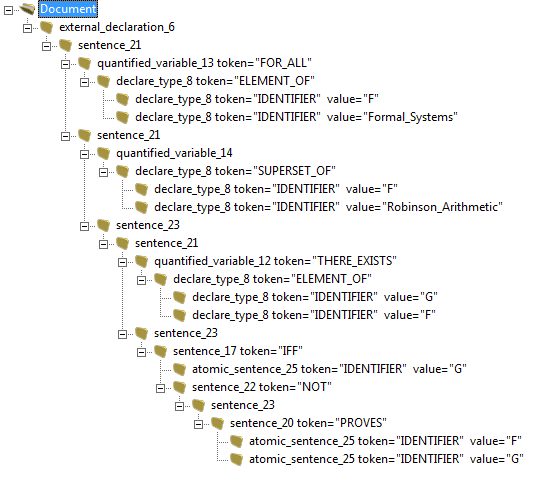# Minimal Type Theory (MTT) parsed output

If the following expression is not satisfied then the conclusion of Kurt Gödel’s 1931 Incompleteness Theorem is incorrect. There is no such statement in F.

“For every Formal_System that is a super set of Robinson_Arithmetic there exists a proposition that is materially equivalent to a statement of its own unprovability.”

∀F ∈ Formal_Systems F ⊇ Robinson_Arithmetic (∃G ∈ F (G ↔ ~(F ⊢ G)))